with this app, you can create your own formula to use, there are 4 vars can be used in this app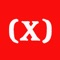Formula Editor

by Peisheng Zhang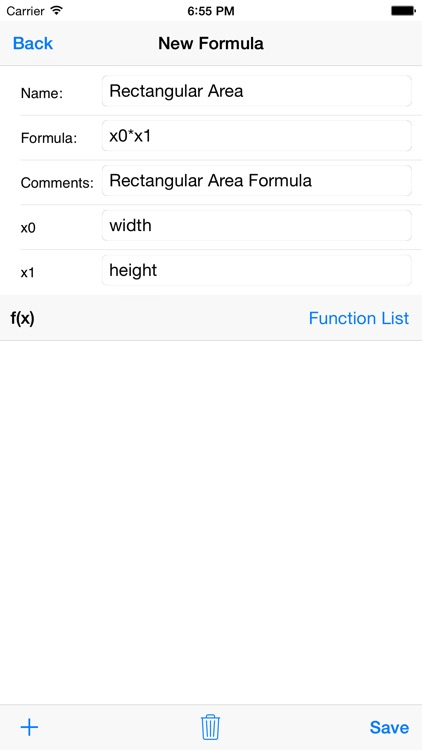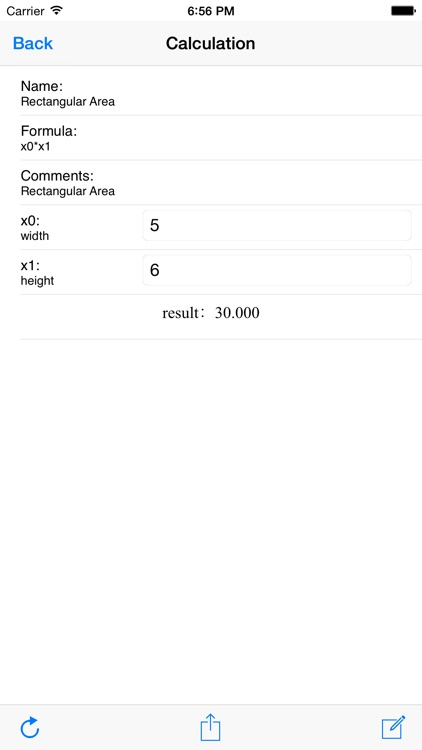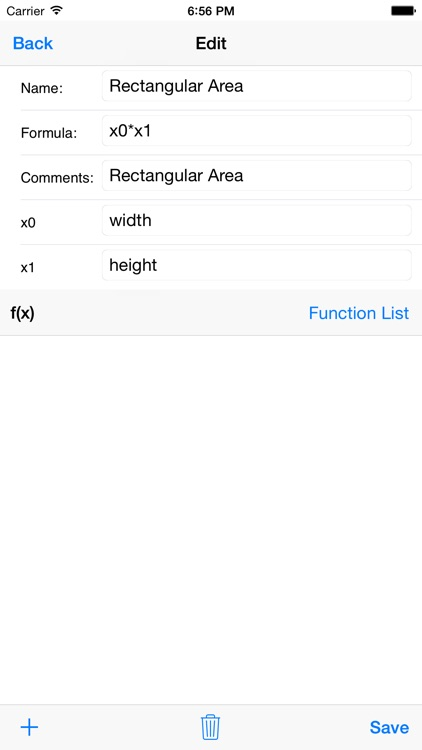with this app, you can create your own formula to use, there are 4 vars can be used in this app. for example, if you want to create a formula for rectangular area, you can do as below:App Details

Version
1.3
Rating
NA
Size
12Mb
Genre
Utilities Productivity
Last updated
May 31, 2016
Release date
February 7, 2015

App Screenshots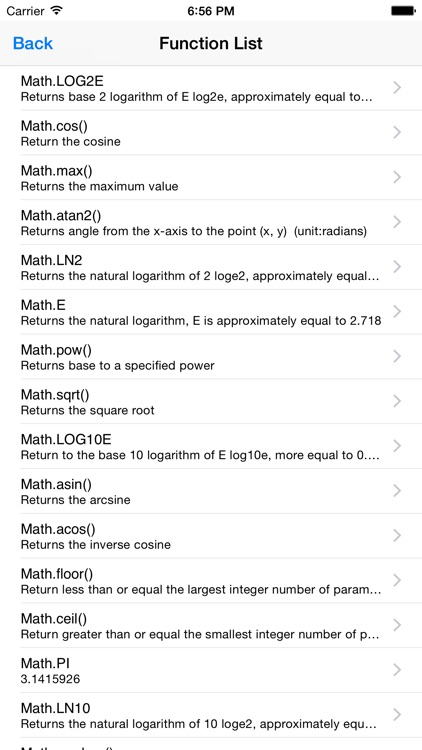App Store Description

with this app, you can create your own formula to use, there are 4 vars can be used in this app. for example, if you want to create a formula for rectangular area, you can do as below:
Creating a formula:
1. create a new formula by click + on tool bar.
2. input formula name, here we input "rectangular area".
3. input formula, here we should input x0*x1.
4. we need two vars here, so click + to add one more var.
5. input var for x0, here we use "width".
6. input var for x1, here we use "height".
7. click save on tool bar, it is done.
8. go back to main page, you will be able to see there is a formula with name rectangular area there.
Calculation
1. click on formula name, it brings you to calculation page.
2. input the vars, should all in number.
3. click the first button on tool bar, you should get result.
Edit
If you find your formula has error, you can edit the formula to modify it.
1. go to formula calculation page, click the edit button on tool bar.
2. edit the formula as you want.
3. there is a function list can be used into your formula, just click the function list, on function list, click the function name, then that function will be input into formula filed.